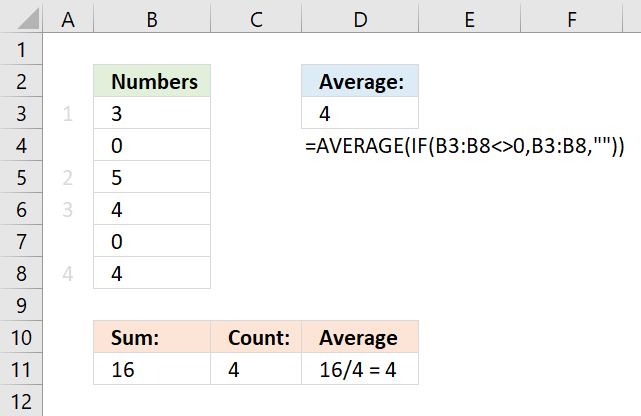Author: Oscar Cronquist Article last updated on December 13, 2017The array formula in cell D3 calculates an average and ignores 0 (zeros).

=AVERAGE(IF(B3:B8<>0,B3:B8,""))

The formula above is an array formula. To enter an array formula, type the formula in a cell then press and hold CTRL + SHIFT simultaneously, now press Enter once. Release all keys.

The formula bar now shows the formula enclosed with curly brackets telling you that you entered the formula successfully. Don't enter the curly brackets yourself.

IF(B3:B8<>0,B3:B8,"")

The IF function checks if values in B3:B8 are not equal to 0, it returns the value if TRUE and returns a blank if it is FALSE.

IF({TRUE;FALSE;TRUE;TRUE;FALSE;TRUE},B3:B8,"")

becomes

IF({TRUE;FALSE;TRUE;TRUE;FALSE;TRUE},{3;0;5;4;0;4},"")

and returns

{3;"";5;4;"";4}

The AVERAGE function then calculates the average of the numbers in the array, it ignores the blank values.

AVERAGE({3;"";5;4;"";4})

returns 4. 3 + 5 +4 +4 = 16. 16/4 = 4.